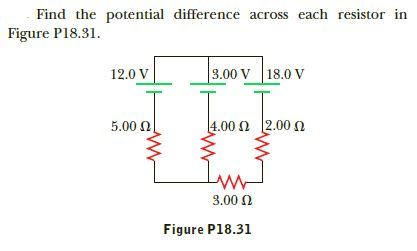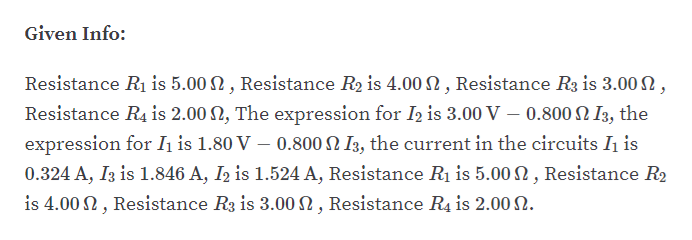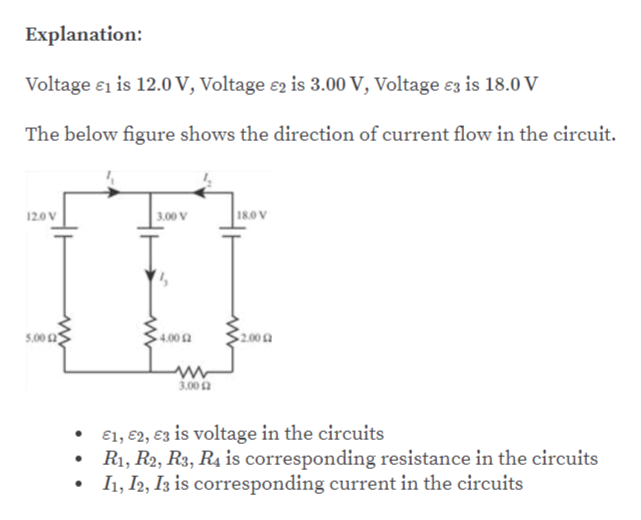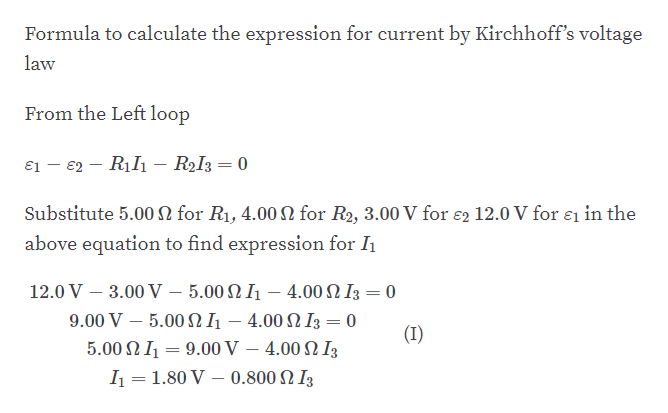# Find the potential difference across each resistor inFigure P18.31.3.00 V18.0 V12.0 V|2.00 Ω1.00 Ω5.00 N3.00 0Figure P18.31

Question
6 viewshelp_outlineImage TranscriptioncloseFind the potential difference across each resistor in Figure P18.31. 3.00 V 18.0 V 12.0 V |2.00 Ω 1.00 Ω 5.00 N 3.00 0 Figure P18.31 fullscreen
check_circle

Step 1help_outlineImage TranscriptioncloseGiven Info: Resistance R1 is 5.00 N , Resistance R2 is 4.00 N , Resistance R3 is 3.00 N, Resistance R4 is 2.00 N, The expression for I2 is 3.00 V – 0.800 N I3, the expression for Ih is 1.80 V – 0.800 N I3, the current in the circuits Ih is 0.324 A, I3 is 1.846 A, I2 is 1.524 A, Resistance Rị is 5.00 N , Resistance R2 is 4.00 N , Resistance R3 is 3.00 N , Resistance R4 is 2.00 N. fullscreen
Step 2help_outlineImage TranscriptioncloseExplanation: Voltage e1 is 12.0 V, Voltage e2 is 3.00 V, Voltage e3 is 18.0 V The below figure shows the direction of current flow in the circuit. 18.0 V 12.0 V 3.00 V 200 5.00 0 4.00 2 3.00a €1, €2, ɛ3 is voltage in the circuits R1, R2, R3, R4 is corresponding resistance in the circuits I1, I2, I3 is corresponding current in the circuits fullscreen
Step 3help_outlineImage TranscriptioncloseFormula to calculate the expression for current by Kirchhoff's voltage law From the Left loop ɛ1 - €2 – R1I1 – RI3 = 0 Substitute 5.00 N for R1, 4.00N for R2, 3.00 V for e2 12.0 V for ɛi in the above equation to find expression for I 12.0 V – 3.00 V – 5.00 N I1 – 4.00 N I3 = 0 9.00 V- 5.00 Ω 1- 4.00 Ω Β-0 (I) 5.00 N1 = 9.00 V – 4.00 N I3 I = 1.80 V – 0.800 N I3 fullscreen

### Want to see the full answer?

See Solution

#### Want to see this answer and more?

Solutions are written by subject experts who are available 24/7. Questions are typically answered within 1 hour.*

See Solution
*Response times may vary by subject and question.
Tagged in
SciencePhysics

### Current Electricity